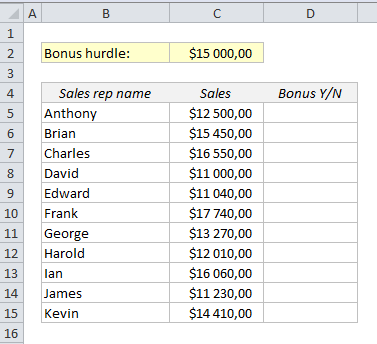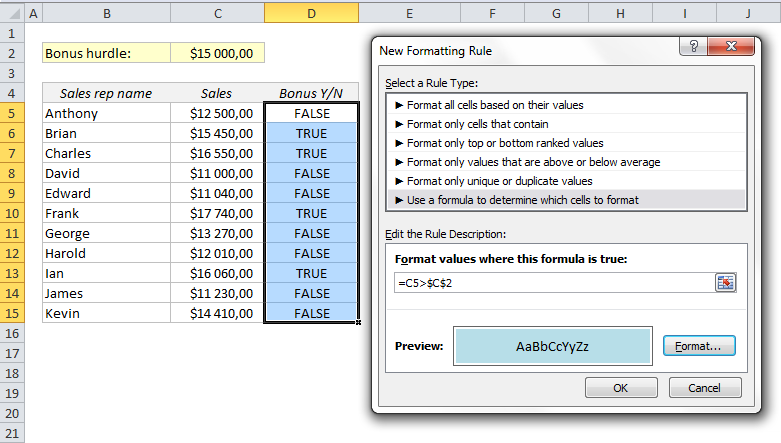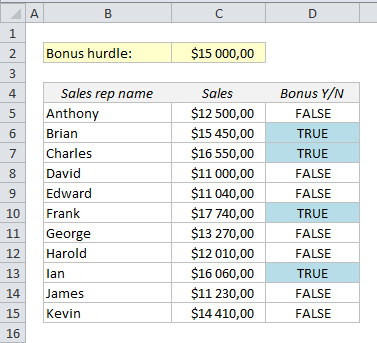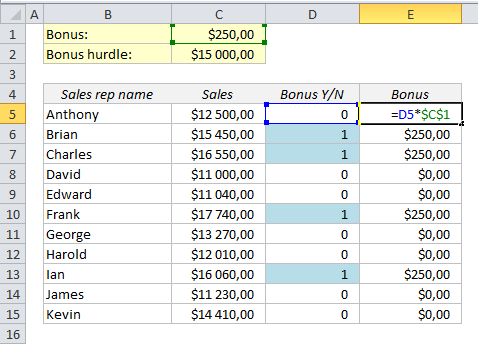# Boolean logic in Excel: TRUE/FALSE instead of IF functions

Most of us use the IF function all the time, for example to test if one value is larger than another value. But many times there is an easier way to do it! Let’s use Boolean logic instead – it will save you a lot of time and struggle. Boolean logic, or Boolean algebra, is a kind of algebra where you’re looking for a TRUE or FALSE result.

Let’s look at an example. In this sales report, the sales reps get a bonus if they reach \$ 15,000 in sales:The most common way to solve this is an IF statement such as IF(C5>\$C\$2,”TRUE”,”FALSE”)=, but we’ll solve this in the realm of Boolean logic, which makes it a lot easier:

=C5>C2

This formula will return TRUE or FALSE. Of course, in order to copy the formula down, we need to lock the reference to the bonus hurdle in C2 with dollar signs (Shortcut: F4):

=C5>\$C\$2

Getting used to this logic is also the secret to mastering conditional formatting. Conditional formatting relies on the TRUE/FALSE logic: if the statement is true, the cell will be formatted.

Let’s try to conditionally format the trues and falses in this table. Select all the cells in the bonus column, click on Conditional Formatting from the Home ribbon and choose New Rule. Choose “Use a formula to determine which cells to format” and type the same formula as we used above into the formula field.Click on the Format button and choose a fill colour or font colour.

That’s all, and here is the result:Bonus tip: If you want to replace TRUE and FALSE with ones and zeroes, it’s done in a second:

Put the logical statement in brackets and add a zero: =(C5>\$C\$2)+0

This could be a good idea if you want to use the results from column D for further calculations:Other easy tricks:

Are you using a non-English version of Excel? Click here for translations of the 100 most common functions.

1.Dugite on said: